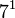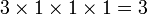# Groups of order 840

## Contents

See pages on algebraic structures of order 840| See pages on groups of a particular order

## Statistics at a glance

The number 840 has the prime factorization:$\! 840 = 2^3 \cdot 3^1 \cdot 5^1 \cdot 7^1 = 8 \cdot 3 \cdot 5 \cdot 7$

There are both solvable and non-solvable groups of this order. There are two possibilities for the composition factors for non-solvable groups:

Quantity Value Explanation
Total number of groups up to isomorphism 186
Number of abelian groups (i.e., finite abelian groups) up to isomorphism 3 (number of abelian groups of order$2^3$) times (number of abelian groups of order$3^1$) times (number of abelian groups of order$5^1$) times (number of abelian groups of order$7^1$) = (number of unordered integer partitions of 3) times (number of unordered integer partitions of 1) times (number of unordered integer partitions of 1) times (number of unordered integer partitions of 1) =$3 \times 1 \times 1 \times 1 = 3$. See classification of finite abelian groups and structure theorem for finitely generated abelian groups.
Number of nilpotent groups (i.e., finite nilpotent group]s) up to isomorphism 5 (number of groups of order 8) times (number of groups of order 3) times (number of groups of order 5) times (number of groups of order 7) =$5 \times 1 \times 1 \times 1 = 5$. See number of nilpotent groups equals product of number of groups of order each maximal prime power divisor, which in turn follows from equivalence of definitions of finite nilpotent group.
Number of supersolvable groups (i.e., finite supersolvable groups) up to isomorphism 164
Number of solvable groups (i.e., finite solvable groups) up to isomorphism 180 There are six non-solvable groups: PLACEHOLDER FOR INFORMATION TO BE FILLED IN: [SHOW MORE]
Number of simple groups 0

## GAP implementation

The order 840 is part of GAP's SmallGroup library. Hence, any group of order 840 can be constructed using the SmallGroup function by specifying its group ID. Also, IdGroup is available, so the group ID of any group of this order can be queried.

Further, the collection of all groups of order 840 can be accessed as a list using GAP's AllSmallGroups function.

Here is GAP's summary information about how it stores groups of this order, accessed using GAP's SmallGroupsInformation function:

gap> SmallGroupsInformation(840);

There are 186 groups of order 840.
They are sorted by their Frattini factors.
1 has Frattini factor [ 210, 1 ].
2 has Frattini factor [ 210, 2 ].
3 has Frattini factor [ 210, 3 ].
4 has Frattini factor [ 210, 4 ].
5 has Frattini factor [ 210, 5 ].
6 has Frattini factor [ 210, 6 ].
7 has Frattini factor [ 210, 7 ].
8 has Frattini factor [ 210, 8 ].
9 has Frattini factor [ 210, 9 ].
10 has Frattini factor [ 210, 10 ].
11 has Frattini factor [ 210, 11 ].
12 has Frattini factor [ 210, 12 ].
13 has Frattini factor [ 420, 13 ].
14 has Frattini factor [ 420, 14 ].
15 has Frattini factor [ 420, 15 ].
16 - 22 have Frattini factor [ 420, 16 ].
23 - 27 have Frattini factor [ 420, 17 ].
28 - 32 have Frattini factor [ 420, 18 ].
33 - 37 have Frattini factor [ 420, 19 ].
38 has Frattini factor [ 420, 20 ].
39 has Frattini factor [ 420, 21 ].
40 has Frattini factor [ 420, 22 ].
41 has Frattini factor [ 420, 23 ].
42 - 48 have Frattini factor [ 420, 24 ].
49 - 55 have Frattini factor [ 420, 25 ].
56 - 62 have Frattini factor [ 420, 26 ].
63 - 69 have Frattini factor [ 420, 27 ].
70 - 76 have Frattini factor [ 420, 28 ].
77 - 83 have Frattini factor [ 420, 29 ].
84 - 90 have Frattini factor [ 420, 30 ].
91 - 93 have Frattini factor [ 420, 31 ].
94 has Frattini factor [ 420, 32 ].
95 has Frattini factor [ 420, 33 ].
96 - 100 have Frattini factor [ 420, 34 ].
101 - 105 have Frattini factor [ 420, 35 ].
106 - 110 have Frattini factor [ 420, 36 ].
111 - 115 have Frattini factor [ 420, 37 ].
116 - 120 have Frattini factor [ 420, 38 ].
121 - 125 have Frattini factor [ 420, 39 ].
126 - 130 have Frattini factor [ 420, 40 ].
131 - 133 have Frattini factor [ 420, 41 ].
134 - 186 have trivial Frattini subgroup.

For the selection functions the values of the following attributes
are precomputed and stored:
IsAbelian, IsNilpotentGroup, IsSupersolvableGroup, IsSolvableGroup,
LGLength, FrattinifactorSize and FrattinifactorId.

This size belongs to layer 2 of the SmallGroups library.
IdSmallGroup is available for this size.